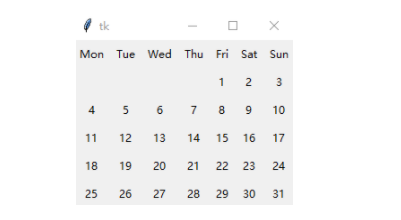python tkinter制作日历界面import calendar
from tkinter import *
root = Tk()
labels = [['Mon','Tue','Wed','Thu','Fri','Sat','Sun']]

MonthCal = calendar.monthcalendar(2020, 5)
for i in range(len(MonthCal)):
labels.append(MonthCal[i])
for r in range(len(MonthCal)+1):
for c in range(7):
if labels[r][c] == 0:
labels[r][c] = ' '
label = Label(root,
text=str(labels[r][c]))
label.grid(row=r,column=c)
root.mainloop()Python开发开发项目